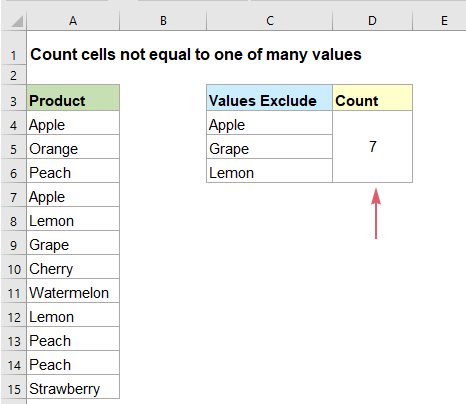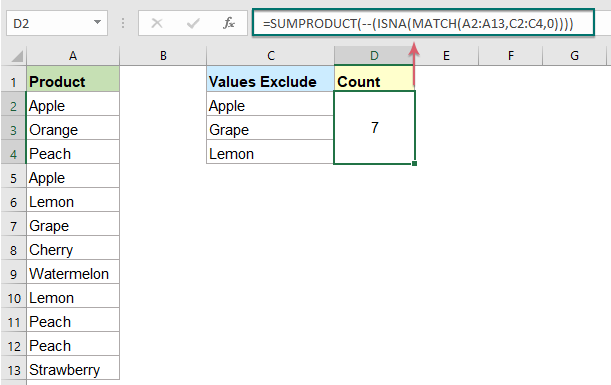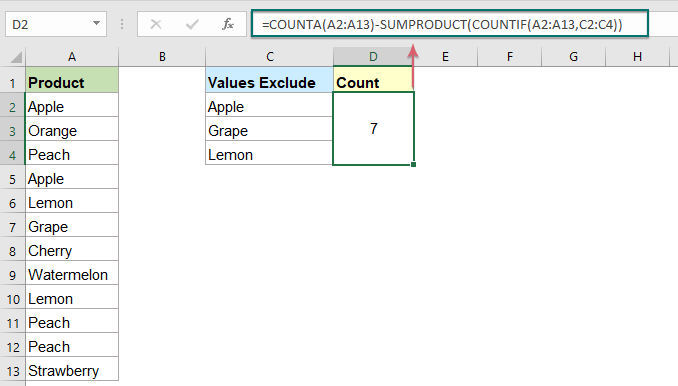## Count number of cells not equal to many values in Excel

In Excel, you may easily get the number of cells not equal to a specific value by using the COUNTIF function, but have you ever tried to count the cells which are not equal to many values? For example, I want to get the total number of the products in column A but exclude the specific items in C4:C6 as below screenshot shown. This article, I will introduce some formulas for solving this job in Excel.#### Count number of cells not equal to many values with formula1

To count cells not equal to many specified values, you can use the combination of the MATCH, ISNA, and SUMPRODUCT functions, the generic syntax is:

= SUMPRODUCT ( -- ( ISNA ( MATCH ( range, exclude_values, 0)))
• range: The list of cells you want to count;
• exclude_values: The values to ignore from the range list.

1. Enter or copy the below formula into a blank cell where you want to output the result:

=SUMPRODUCT(--(ISNA(MATCH(A2:A13,C2:C4,0))))

2. Then, press Enter key to get the correct result, see screenshot:##### Explanation of the formula:
• MATCH(A2:A13,C2:C4,0): This MATCH function returns the position of each matching values as a number, and returns #N/A error value where not found. It means, when the values in A2:A13 equal to the values in C2:C4, it will return a number, otherwise, it returns an #NA error. Here the result is: {1;#N/A;#N/A;1;3;2;#N/A; #N/A;3;#N/A; #N/A; #N/A;}.
• ISNA(MATCH(A2:A13,C2:C4,0))= ISNA({1;#N/A;#N/A;1;3;2;#N/A; #N/A;3;#N/A; #N/A; #N/A;}): This ISNA function forces the error values to become TRUE, and the numbers to become FASLE, so you will get this: {FALSE;TRUE;TRUE;FALSE;FALSE;FALSE;TRUE;TRUE;FALSE;TRUE;TRUE;TRUE}.
• --(ISNA(MATCH(A2:A13,C2:C4,0))))=--({FALSE;TRUE;TRUE;FALSE;FALSE;FALSE;TRUE;TRUE;FALSE;TRUE;TRUE;TRUE}): The -- double negative operator converts all TRUEs to 1s and FALSEs to 0s as this: SUMPRODUCT(--(ISNA(MATCH(A2:A13,C2:C4,0))))=SUMPRODUCT({0;1;1;0;0;0;1;1;0;1;1;1;}).
• And then the SUMPRODUCT function is used to sum the numbers in the array and will get the result:7.

#### Count number of cells not equal to many values with formula2

To solve this job in Excel, you can also apply the combination of the COUNTA, SUMPRODUCT and COUNTIF functions, the generic syntax is:

=COUNTA(range)-SUMPRODUCT(COUNTIF(range,exclude_values))
• range: The list of cells you want to count;
• exclude_values: The values to ignore from the range list.

1. Please enter or copy the below formula into a blank cell to put the result:

=COUNTA(A2:A13)-SUMPRODUCT(COUNTIF(A2:A13,C2:C4))

2. Then, press Enter key to get the result as following screenshot shown:##### Explanation of the formula:
• COUNTA(A2:A13): This COUNTA function returns the number of all non-empty cells in range A2:A13. And it will get the result:12.
• COUNTIF(A2:A13,C2:C4): This COUNTIF function counts the cells in range A2:A13 that are equal to the criterion in C2:C4, so, you will get this result: {2;1;2} which means Apple appears twice, Grape appears once and Lemon appears twice.
• SUMPRODUCT(COUNTIF(A2:A13,C2:C4))=SUMPRODUCT({2;1;2}): This SUMPRODUCT function sums all items in the array and returns a final result:5.
• COUNTA(A2:A13)-SUMPRODUCT(COUNTIF(A2:A13,C2:C4))=12-5 :The result (5) of the SUMPRODUCT function is subtracted from the count of all non-empty cells (12) to get the final result you need.

Note: Both the above formulas are not case sensitive.

#### Relative function used:

• MATCH:
• The MATCH function searches for a specific value in a range of cells, and returns the relative position of this value.
• ISNA:
• The ISNA function is use to check if a formula returns a #N/A error.
• SUMPRODUCT:
• The SUMPRODUCT function can be used to multiply two or more columns or arrays together, and then get the sum of products.
• COUNTIF:
• The COUNTIF function is a statistical function in Excel which is used to count the number of cells that meet a criterion.
• COUNTA:
• The COUNTA function returns the number of cells excepted empty cells.

#### More articles:

• Count Number Of Cells Equals To One Of Many Values In Excel
• Supposing, I have a list of products in column A, now, I want to get the total number of specific products Apple, Grape and Lemon which listed in range C4:C6 from column A as below screenshot shown. Normally, in Excel, the simple COUNTIF and COUNTIFS functions will not work in this scenario. This article, I will talk about how to solve this job quickly and easily with the combination of SUMPRODUCT and COUNTIF functions.
• Count Number Of Cells Not Between Two Given Numbers In Excel
• To count the number of cells between two numbers is a common task for us in Excel, but, in certain case, you may want to count the cells not between two given numbers. For example, I have a product list with the sales from Monday to Sunday, now, I need to get the number of cells which not between the specific low and high numbers as below screenshot shown. This article, I will introduce some formulas for dealing with this task in Excel.

### The Best Office Productivity Tools

#### Kutools for Excel - Helps You To Stand Out From Crowd

 Popular Features: Find, Highlight or Identify Duplicates  |  Delete Blank Rows  |  Combine Columns or Cells without Losing Data  |  Round without Formula ... Super VLookup: Multiple Criteria  |  Multiple Value  |  Across Multi-Sheets  |  Fuzzy Lookup... Adv. Drop-down List: Easy Drop Down List  |  Dependent Drop Down List  |  Multi-select Drop Down List... Column Manager: Add a Specific Number of Columns  |  Move Columns  |  Toggle Visibility Status of Hidden Columns  |  Compare Columns to Select Same & Different Cells ... Featured Features: Grid Focus  |  Design View  |  Big Formula Bar  |  Workbook & Sheet Manager | Resource Library (Auto Text)  |  Date Picker  |  Combine Worksheets  |  Encrypt/Decrypt Cells  |  Send Emails by List  |  Super Filter  |  Special Filter (filter bold/italic/strikethrough...) ... Top 15 Toolsets:  12 Text Tools (Add Text, Remove Characters ...)  |  50+ Chart Types (Gantt Chart ...)  |  40+ Practical Formulas (Calculate age based on birthday ...)  |  19 Insertion Tools (Insert QR Code, Insert Picture from Path ...)  |  12 Conversion Tools (Numbers to Words, Currency Conversion ...)  |  7 Merge & Split Tools (Advanced Combine Rows, Split Excel Cells ...)  |  ... and more

Kutools for Excel Boasts Over 300 Features, Ensuring That What You Need is Just A Click Away...#### Office Tab - Enable Tabbed Reading and Editing in Microsoft Office (include Excel)

• One second to switch between dozens of open documents!
• Reduce hundreds of mouse clicks for you every day, say goodbye to mouse hand.
• Increases your productivity by 50% when viewing and editing multiple documents.
• Brings Efficient Tabs to Office (include Excel), Just Like Chrome, Edge and Firefox.